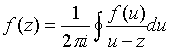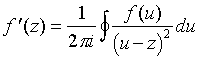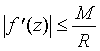## Non-elementary proofs of the Fundamental Theorem of Algebra

The Fundamental Theorem of Algebra is deeply woven into the fabric of many aspects of mathematics, from which have emerged proofs with greatly differing viewpoints. Two such proofs, which are particularly well-known, are described briefly below, with references where details can be found.

## Sketch of Proof by the methods of the theory of Complex Variables (after Liouville)

It is proved in the theory of Complex Variables that for a differentiable function fCC,where the integral is taken over a suitable closed curve enclosing a region where f and its derivatives are defined and continuous. By means of careful limiting arguments, it follows that the values of a differentiable function on a suitable region of C are determined by its values on the boundary of the region, according to the Cauchy Integral Formula:.

From this formula, a similar formula for the first derivative may be obtained:From this integral formula, one obtains the Cauchy Estimate for the first derivative: if |f(z)| < M on the closed disc centered on z with radius R, thenFrom this estimate, one obtains Liouville’s Theorem: a bounded function differentiable on the entire complex plane is constant.

Now consider a non-constant polynomial P(z) of degree n, and suppose it has no roots. Then the reciprocal 1/P(z) is a continuous, differentiable function on the entire complex plane. Since, as we have shown above, if P(z) has no roots, |P(z)| takes on a global minimum value, 1/|P(z)| is bounded. By Liouville’s Theorem 1/P(z), and hence P(z), must be a constant, contradicting our choice of P(z). This proves the Fundamental Theorem of Algebra. (See, for example, Boas, RP, Invitation to Complex Analysis, Random House, New York, 1987, for details.)